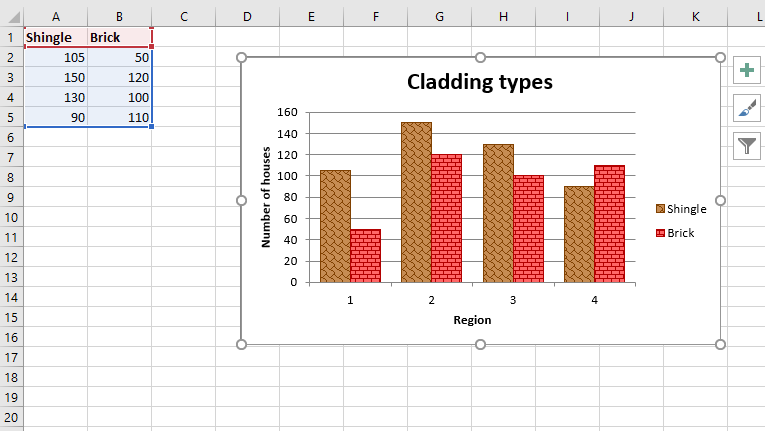# Python | Plotting an Excel chart with pattern fills in column using XlsxWriter module

• Difficulty Level : Medium
• Last Updated : 30 Jan, 2022

Prerequisite: Create and Write on an excel sheet
XlsxWriter is a Python library using which one can perform multiple operations on excel files like creating, writing, arithmetic operations and plotting graphs. Let’s see how to plot chart with pattern fills, using realtime data.
Charts are composed of at least one series of one or more data points. Series themselves are comprised of references to cell ranges. For plotting the charts on an excel sheet, firstly, create chart object of specific chart type( i.e Column chart etc.). After creating chart objects, insert data in it and lastly, add that chart object in the sheet object.
Code : Plot a Chart with pattern fills.
For plotting this type of chart on an excel sheet, use add_series() method with ‘pattern’ keyword argument of the chart object.

## Python3

 `# import xlsxwriter module``import` `xlsxwriter` `# Workbook() takes one, non-optional, argument  ``# which is the filename that we want to create.``workbook ``=` `xlsxwriter.Workbook(``'chart_pattern.xlsx'``)` `# The workbook object is then used to add new  ``# worksheet via the add_worksheet() method. ``worksheet ``=` `workbook.add_worksheet()` `# Create a new Format object to formats cells``# in worksheets using add_format() method .``  ` `# here we create bold format object .``bold ``=` `workbook.add_format({``'bold'``: ``1``})` `# Add the worksheet data that the charts will refer to.``headings ``=` `[``'Shingle'``, ``'Brick'``]``data ``=` `[``    ``[``105``, ``150``, ``130``, ``90` `],``    ``[``50``,  ``120``, ``100``, ``110``],``]` `# Write a row of data starting from 'A1'``# with bold format .``worksheet.write_row(``'A1'``, headings, bold)` `# Write a column of data starting from ``# 'A2', 'B2' respectively .``worksheet.write_column(``'A2'``, data[``0``])``worksheet.write_column(``'B2'``, data[``1``])` `# Create a chart object that can be added``# to a worksheet using add_chart() method.` `# here we create a column chart object``chart ``=` `workbook.add_chart({``'type'``: ``'column'``})` `# Add a data series with pattern to a chart``# using add_series method.  The gap is used``# to make the patterns more visible.``  ` `# Configure the first series.``# = Sheet1 !\$A\$1 is equivalent to ['Sheet1', 0, 0].` `# note : spaces is not inserted in b / w``# = and Sheet1, Sheet1 and !``# if space is inserted it throws warning.``chart.add_series({``    ``'name'``:   ``'= Sheet1 !\$A\$1'``,``    ``'values'``: ``'= Sheet1 !\$A\$2:\$A\$5'``,``    ``'pattern'``: {``        ``'pattern'``:  ``'shingle'``,``        ``'fg_color'``: ``'# 804000'``,``        ``'bg_color'``: ``'# c68c53'``    ``},``    ``'border'``:  {``'color'``: ``'# 804000'``},``    ``'gap'``:     ``70``,``})` `# Configure a second series.``chart.add_series({``    ``'name'``:   ``'= Sheet1 !\$B\$1'``,``    ``'values'``: ``'= Sheet1 !\$B\$2:\$B\$5'``,``    ``'pattern'``: {``        ``'pattern'``:  ``'horizontal_brick'``,``        ``'fg_color'``: ``'# b30000'``,``        ``'bg_color'``: ``'# ff6666'``    ``},``    ``'border'``:  {``'color'``: ``'# b30000'``},``})` `# Add a chart title``chart.set_title ({``'name'``: ``'Cladding types'``})`  `# Add x-axis label``chart.set_x_axis({``'name'``: ``'Region'``})` `# Add y-axis label``chart.set_y_axis({``'name'``: ``'Number of houses'``})` `# add chart to the worksheet with given``# offset values at the top-left corner of``# a chart is anchored to cell D2 .``worksheet.insert_chart(``'D2'``, chart,  {``'x_offset'``: ``25``, ``'y_offset'``: ``10``})` `# Finally, close the Excel file ``# via the close() method. ``workbook.close()`

Output :My Personal Notes arrow_drop_up